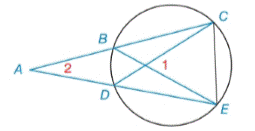Chapter 6.2, Problem 17EElementary Geometry For College St...

7th Edition
Alexander + 2 others
ISBN: 9781337614085

Solutions

Chapter
SectionElementary Geometry For College St...

7th Edition
Alexander + 2 others
ISBN: 9781337614085
Textbook Problem

Given: m ∠ 1 = 62 ° m ∠ 2 = 26 ° Find: m C E ⌢ and m B D ⌢Exercises 16, 17

To determine

To find:

mCE and mBD when m1=62°,m2=26°

Explanation

mCE=xmBD=y

Theorem:

The measure of an angle formed by two chords that intersect within a circle is one-half the sum of the measures of the arcs intercepted by the angle and its vertical angle.

mCE and mBD when m1=62°,m2=26°

By Theorem

m1=12(mCE+mBD)62°=12(x+y)x+y=2(62°)x+y=124°

Theorem:

The measure of an angle formed when two secants intersect at a point outside the circle is one-half the difference of the measures of the two intercepted arc

Still sussing out bartleby?

Check out a sample textbook solution.

See a sample solution

The Solution to Your Study Problems

Bartleby provides explanations to thousands of textbook problems written by our experts, many with advanced degrees!

Get Started

Evaluate the integrals in Problems 1-26.

Mathematical Applications for the Management, Life, and Social Sciences

Exponential Form Write the equation in exponential form. 23. log x = y

Precalculus: Mathematics for Calculus (Standalone Book)

Subtract and check: 75612397_

Elementary Technical Mathematics

For 0

Study Guide for Stewart's Multivariable Calculus, 8th

The area of the shaded region is given by:

Study Guide for Stewart's Single Variable Calculus: Early Transcendentals, 8th